# DAV Class 3 Maths Chapter 5 Worksheet 2 Solutions

The DAV Class 3 Maths Book Solutions and DAV Class 3 Maths Chapter 5 Worksheet 2 Solutions of Division offer comprehensive answers to textbook questions.

## DAV Class 3 Maths Ch 5 WS 2 Solutions

Divide using repeated subtraction.

Question 1.
14 by 7
Solution:14 ÷ 7 = 2

Question 2.
30 by 5
Solution: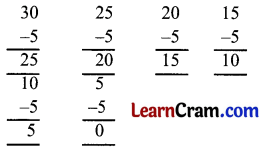30 ÷ 5 = 6Question 3.
27 by 9
Solution: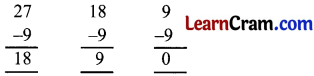27 ÷ 9 = 3

Question 4.
48 by 8
Solution: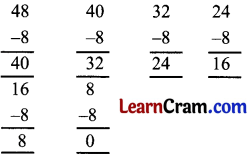48 ÷ 8 = 6Question 5.
60 by 10
Solution: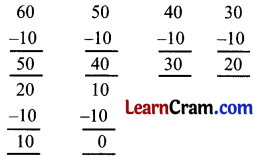60 ÷ 10 = 6

Question 6.
72 by 8
Solution: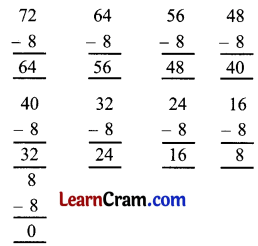72 ÷ 8 = 9### DAV Class 3 Maths Chapter 5 Worksheet 2 Notes

Distribute 16 mangoes among 4 children.
Distribute equally to each child so that each get 4 mangoes.
1. Ram takes 4 mangoes = 16 – 4 = 122. Manu takes 4 mangoes = 12 – 4 = 8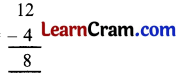3. Ritu takes 4 mangoes = 8 – 4 = 44. Ramesh takes 4 mangoes = 4 – 4 = 04 Children get 4 mangoes each
16 ÷ 4 = 4
4 is quotient